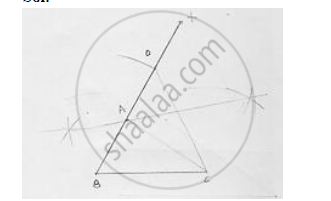# Construct a δAbc in Which Bc = 3.6 Cm, Ab + Ac = 4.8 Cm and ∠B = 60°. - Mathematics

Construct a ΔABC in which BC = 3.6 cm, AB + AC = 4.8 cm and ∠B = 60°.

#### SolutionSteps of construction:
1. Draw a line segment BC of 3.6cm.
2. At the point B, draw ∠xBC  of 60°
3. With center B and radius 4.8cm, draw an arc which intersects XB at D.
4. Join DC
5. Draw the perpendicular bisector of DC which intersects DB at A.
6. Join AC
Hence ΔABCis the required triangle

Concept: Some Constructions of Triangles
Is there an error in this question or solution?

#### APPEARS IN

RD Sharma Mathematics for Class 9
Chapter 16 Constructions
Exercise 16.3 | Q 1 | Page 18

Share